CBSE Class 10 Sample Paper for 2021 Boards - Maths Standard

Class 10
Solutions of Sample Papers for Class 10 Boards

## Water is flowing through a cylindrical pipe of internal diameter 2cm, into a cylindrical tank of base radius 40 cm at the rate of 0.7m/sec. By how much will the water rise in the tank in half an hour?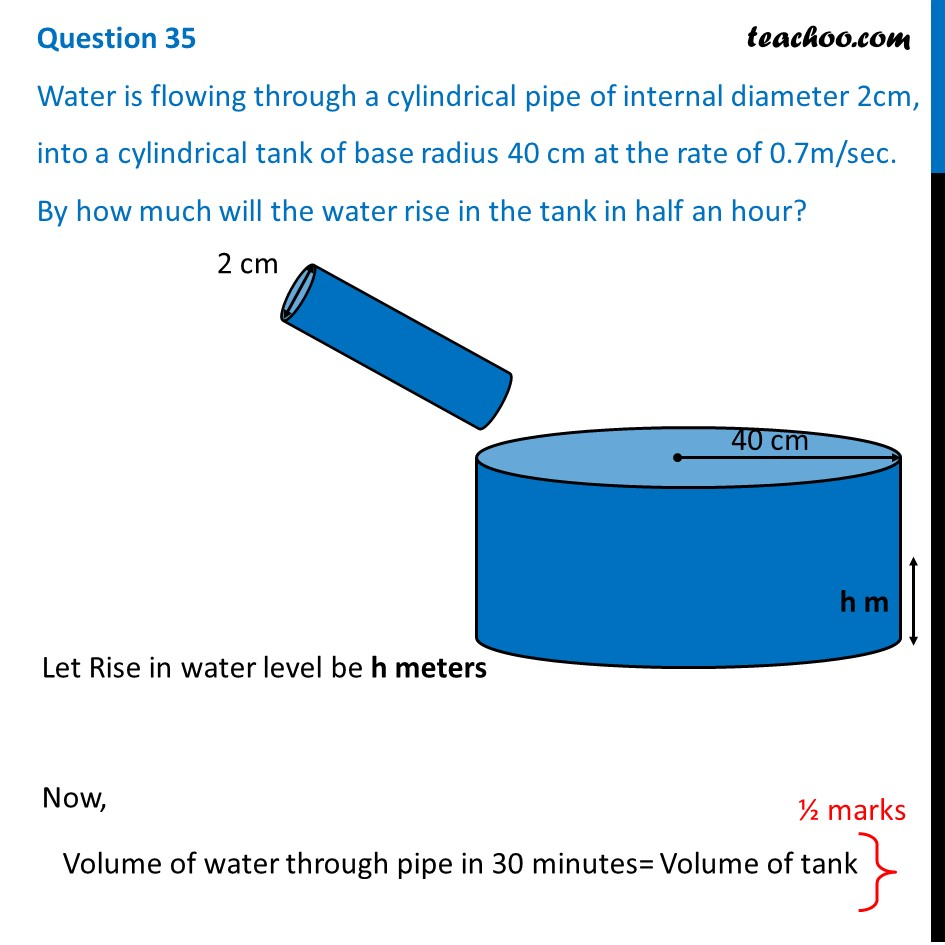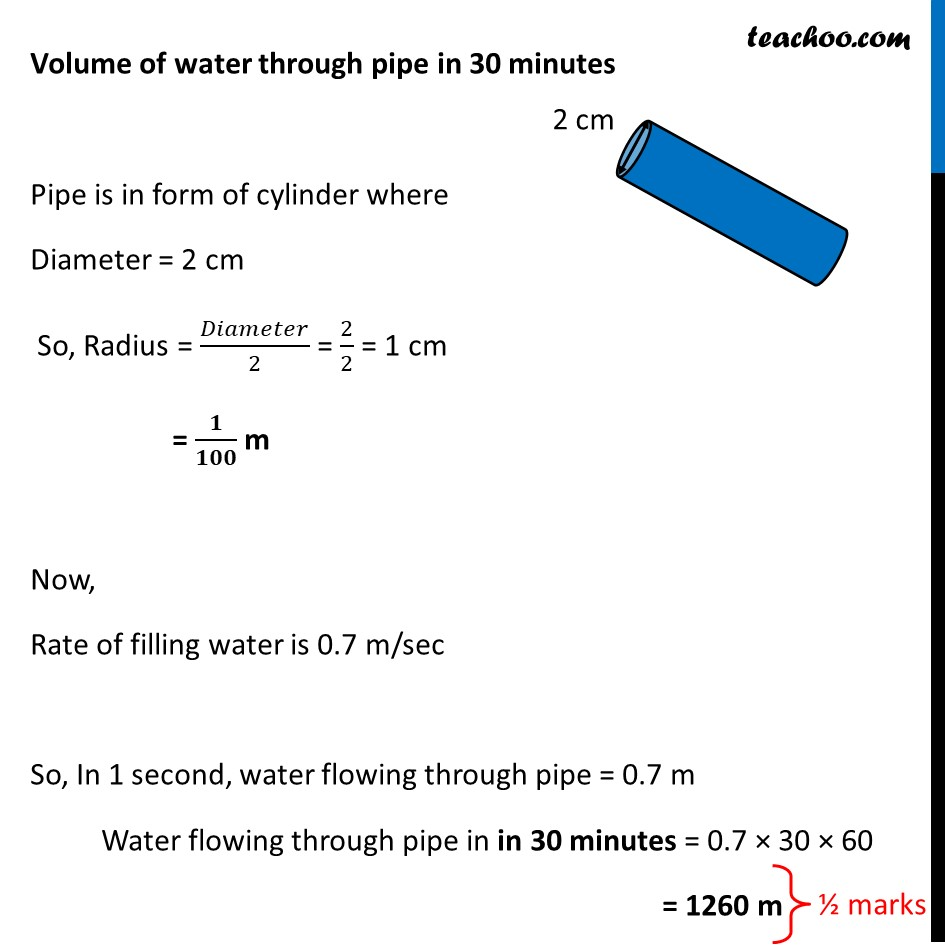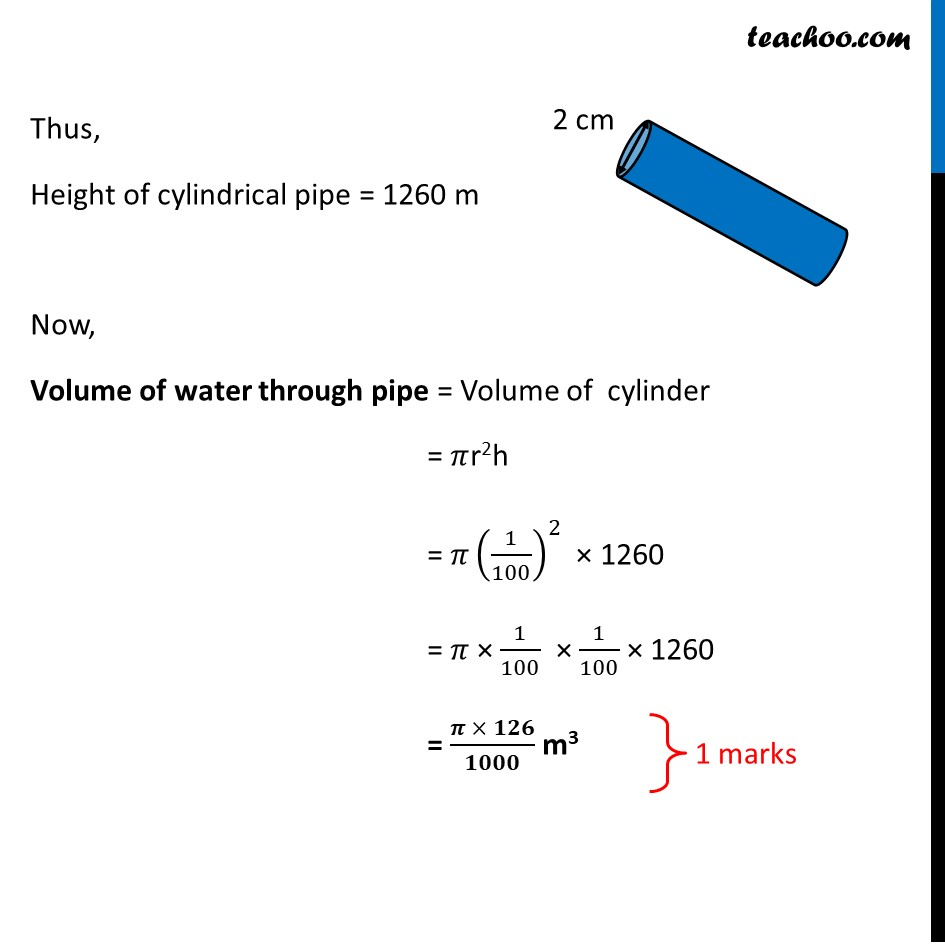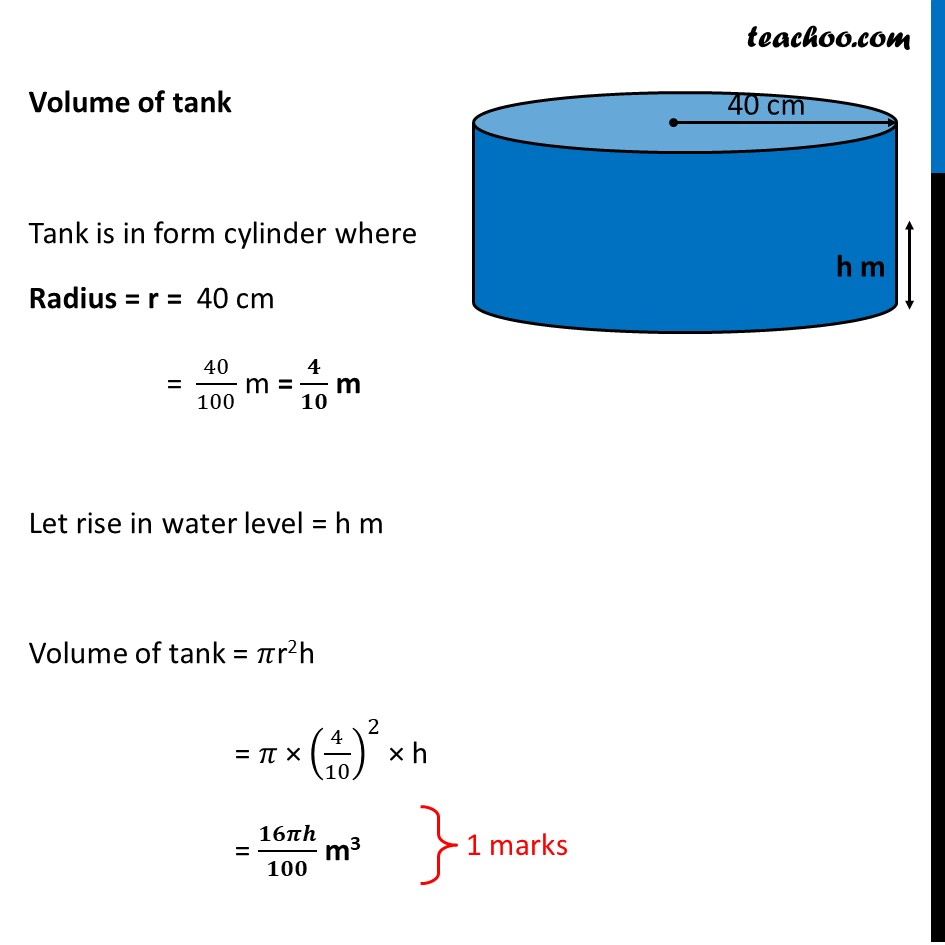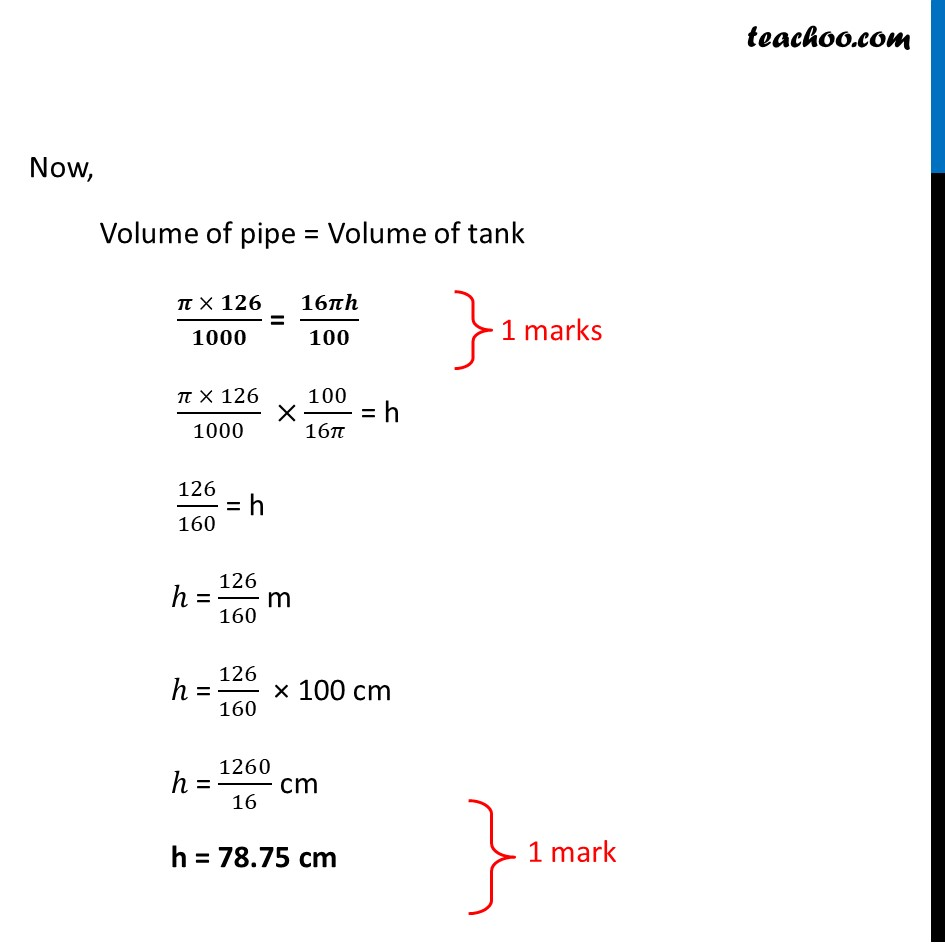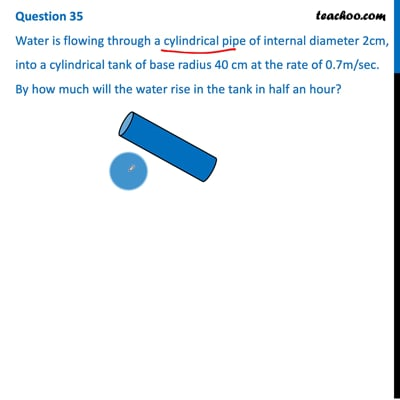This video is only available for Teachoo black users

Introducing your new favourite teacher - Teachoo Black, at only ₹83 per month

### Transcript

Let Rise in water level be h meters Now, Volume of water through pipe in 30 minutes= Volume of tank Volume of water through pipe in 30 minutes Pipe is in form of cylinder where Diameter = 2 cm So, Radius = 𝐷𝑖𝑎𝑚𝑒𝑡𝑒𝑟/2 = 2/2 = 1 cm = 𝟏/𝟏𝟎𝟎 m Now, Rate of filling water is 0.7 m/sec So, In 1 second, water flowing through pipe = 0.7 m Water flowing through pipe in in 30 minutes = 0.7 × 30 × 60 = 1260 m Thus, Height of cylindrical pipe = 1260 m Now, Volume of water through pipe = Volume of cylinder = 𝜋r2h = 𝜋(1/100)^2 × 1260 = 𝜋 × 1/100 × 1/100 × 1260 = (𝝅 × 𝟏𝟐𝟔)/𝟏𝟎𝟎𝟎 m3 Volume of tank Tank is in form cylinder where Radius = r = 40 cm = 40/100 m = 𝟒/𝟏𝟎 m Let rise in water level = h m Volume of tank = 𝜋r2h = 𝜋 × (4/10)^2 × h = 𝟏𝟔𝝅𝒉/𝟏𝟎𝟎 m3 Now, Volume of pipe = Volume of tank (𝝅 × 𝟏𝟐𝟔)/𝟏𝟎𝟎𝟎 = 𝟏𝟔𝝅𝒉/𝟏𝟎𝟎 (𝜋 × 126)/1000 ×100/(16𝜋 ) = h 126/160 = h ℎ = 126/160 m ℎ = 126/160 × 100 cm ℎ = 1260/16 cm h = 78.75 cm